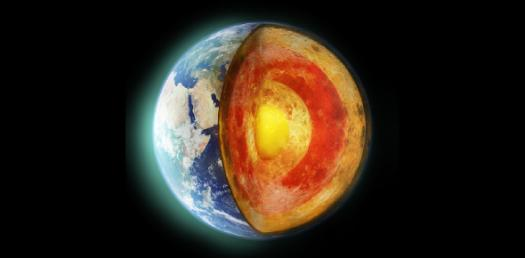# Earth Science - Chapter 8 (Earthquakes)

98 Questions | Total Attempts: 248SettingsCreate your own QuizChapters 8

• 1.
Who first explained mechanism for earthquakes?
• A.

H. Reid

• B.

M. Reid

• C.

H. Richter

• D.

M. Richter

• 2.
Earthquakes are
• A.

Movements along easements

• B.

Movements along faults

• C.

Movements along rock formations

• D.

Movements along pipelines

• 3.
Earthquakes are produced by
• A.

Avalanches

• B.

Rock formations

• C.

Rapid release of energy

• D.

Breaks in the earth

• 4.
Movement along faults are explained by
• A.

Elastic rebound theory

• B.

Vibration theory

• C.

Fault tectonics theory

• D.

Plate tectonics theory

• 5.
Seismology is
• A.

The study of earthquake waves

• B.

The study of earthquake faults

• C.

The study of earthquake energy

• D.

The study of elastic rebound

• 6.
A general feature of an earthquake is
• A.

Associated with movements along faults

• B.

Vibration of the Earth produced by the rapid release of energy

• C.

A & B

• D.

None of the above

• 7.
Rocks spring back is a phenomena called
• A.

Elastic Rebound

• B.

Plate Tectonics

• C.

Seismology

• D.

None of the above

• 8.
A general feature of an earthquake is
• A.

Vibration of Earth produced by the rapid release of energy

• B.

Preceded by foreshocks and followed by afterschocks

• C.

Movements along faults

• D.

All of the above

• 9.
Which is an earthquake recording instrument?
• A.

Barometer

• B.

Richter scale

• C.

Seismograph

• D.

None of the above

• 10.
Which instrument records the movement of Earth?
• A.

Seismograph

• B.

Seismogram

• C.

Barometer

• D.

Richter scale

• 11.
A seismogram is ...
• A.

A record of the Earth's movement

• B.

An instrument that records the Earth's movement

• C.

A measure of the energy of the Earth

• D.

A measure of the Earth's faults

• 12.
A seismogram records ...
• A.

Primary vs. secondary waves

• B.

The strength of the earthquake

• C.

Wave amplitude vs. time

• D.

Wave amplitude vs. length

• 13.
What are the main types of earthquake waves?
• A.

Body waves, surface waves

• B.

Primary waves, secondary waves

• C.

Short waves, long waves

• D.

Slow waves, fast waves

• 14.
Surface waves are ...
• A.

Simple in motion and the slowest velocity of all waves

• B.

Simple in motion and the greatest velocity of all waves

• C.

Complex in motion and the greatest velocity of all waves

• D.

Complex in motion and the slowest velocity of all waves

• 15.
Which is true about a surface wave?
• A.

Has complex motion

• B.

Greatest velocity of all waves

• C.

Push-pull (compressional) motion

• D.

Travels through solids, liquids and gases

• 16.
Which waves are made up of primary and secondary waves?
• A.

Surface waves

• B.

Body waves

• C.

Velocity waves

• D.

Solid waves

• 17.
Which is true about primary waves?
• A.

Have a "shake" motion

• B.

Travel only through solids

• C.

Have slower velocity than secondary waves

• D.

None of the above

• 18.
Which is true about primary waves?
• A.

Travel through solids, liquids and gases

• B.

Have a "shake" motion

• C.

Travel only through solids

• D.

Slower velocity than secondary waves

• 19.
Which is true about primary waves?
• A.

Push-pull (compressional) motion

• B.

Travel through gases only

• C.

Slowest velocity of all waves

• D.

All of the above

• 20.
Primary and secondary waves are ....
• A.

Body waves

• B.

Surface waves

• C.

Vibration waves

• D.

Velocity waves

• 21.
Which is true about secondary waves?
• A.

Push-pull (compression) motion

• B.

Travels through solids, liquids and gases

• C.

Slower velocity than primary waves

• D.

None of the above

• 22.
Which is true about primary waves?
• A.

Greatest velocity of all earthquake waves

• B.

Slowest velocity of all earthquake waves

• C.

Shortest of all earthquake waves

• D.

None of the above

• 23.
Which is true about secondary waves?
• A.

"Shake" motion

• B.

Travel only through solids

• C.

Slower velocity than primary (P) waves

• D.

All of the above

• 24.
The place within the Earth where the earthquake originates is the ...
• A.

Focus

• B.

Diameter

• C.

Epicenter

• D.

Magnitude

• 25.
The epicenter is the ...
• A.

Place where the earthquake originates

• B.

Recording of the earthquake's movement

• C.

Point on the surface directly above the focus

• D.

Distance of the earthquake

Related TopicsBack to top
×

Wait!
Here's an interesting quiz for you.